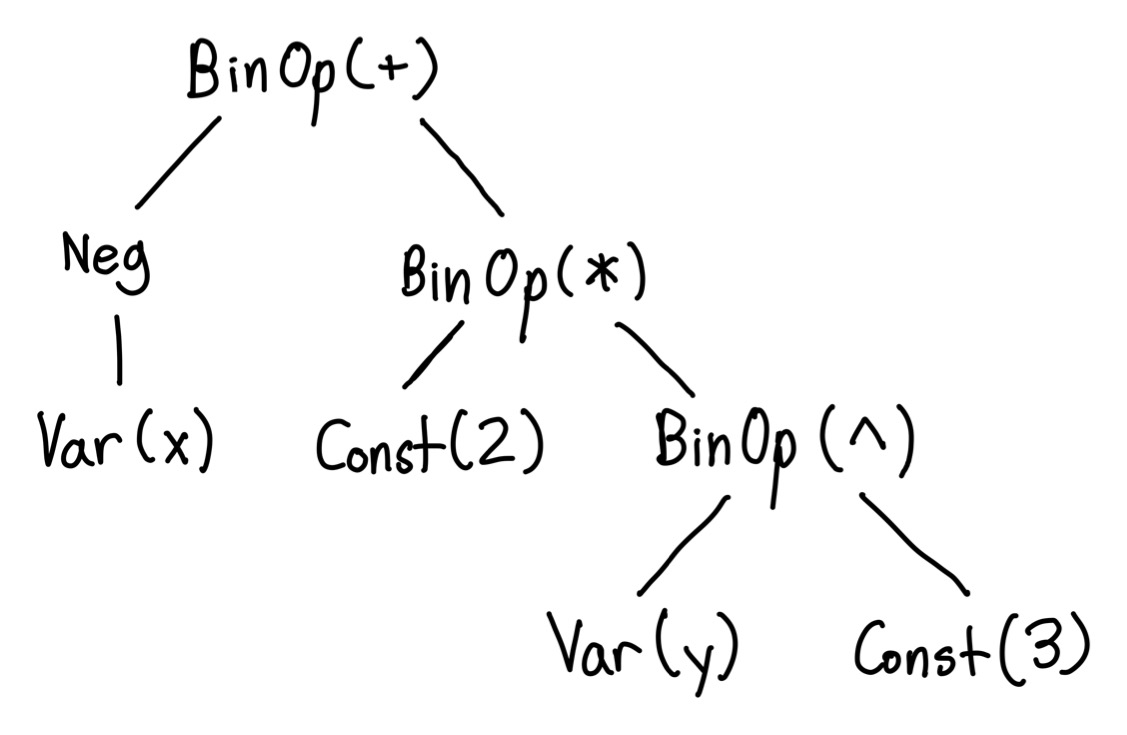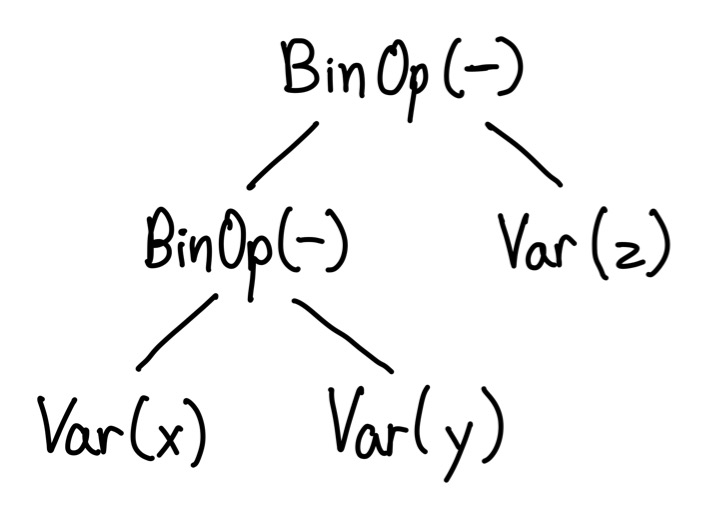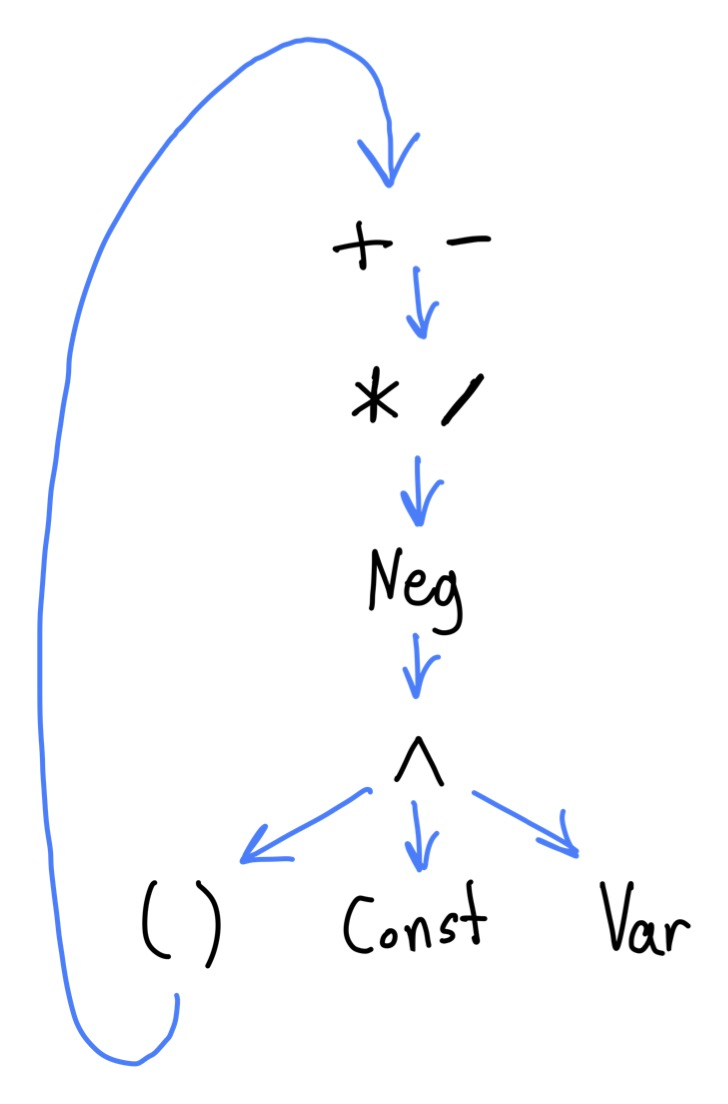# Evaluating Algebraic Expressions using Parser Combinators

Wednesday, August 23rd 2023

In my previous post, I introduced the concept of parser combinators. In this post, we’ll use parser combinators to parse and evaluate algebraic expressions, for example of the form `-x + (2 * y^3)`. We’ll see how parser combinators can handle recursion, operator precedence, and operator associativity.

We will work in steps. First, we’ll define an AST and an evaluator. We will then tokenize the language and finally parse it.

This blog post contains interactive code cells powered by blog-cells. Each cell is an ES module - use the `export` keyword to define values that can be accessed by other cells. Other cells can access exported values using the `\$` global.

## Defining the AST

An AST (abstract syntax tree) is a tree representation of a program. In this case, our AST will represent the individual mathematical operations necessary to evaluate an expression. Here’s an example tree that represents the expression `-x + 2 * y^3`.In our AST, each node can only be evaluated after it’s children are evaluated. This means that computation flows from the bottom (the leaf nodes) up through the tree and eventually to the top, where we receive the final result.

Note that the AST encodes operator precedence. Higher priority operators are further down in the tree, and thus get evaluated before lower priority operators.

Similarly, the AST also encodes associativity. For a left associative operator, operations on the left will be lower on the tree. For example, here is the AST for `x - y - z`.Let’s go ahead and define the node types in our AST. We’ll use a simple validator system to make sure fields always point to what we expect.

## Creating an Evaluator

Implementing evaluation is pretty simple. First we will create a context object that contains mappings from variables to values, for example `{x: 3}`. Then, we recursively traverse our AST, passing down the context object.

## Tokenization

Now that we can evaluate expressions in tree form, our next goal is to turn string representations into trees. We will do this in two steps: tokenization and parsing.

Our tokenizer will consist of a list of regular expressions. Each regular expression will match one type of token, starting at the beginning of the string.

We will repeatedly apply these regular expressions, advancing forward until we have consumed the entire string.

## Parsing with Combinators

In the previous tutorial we simply matched or rejected a string. This time, we want to actually build up an AST representation. To do this, we will add a “value” field to our success result, which will contain the AST node that we just parsed.

Next, we’ll create a base parser that matches a single token. Unlike the `word` parser from the previous tutorial, our `token` parser returns a value - the token that it matched.

I’m also going to pull in the combinators we defined in the previous tutorial. The only change is that these combinators will now return a value on success.

In the case of `sequence`, this is a list of the values of each sub-parser. In the case of of `anyOf`, this is the value of the first successful sub-parser.

### Constructing AST Nodes

We still haven’t constructed any AST nodes - our `token` parser just returns the token that it matched. We can fix this by introducing a `map` function. This function transforms the result of a parser by applying a function - we will use it to create parsers that return AST nodes.

### Recursive Parsing

Let’s try to implement a parser for binary operations. This parser needs to be recursive - binary operations can point to other binary operations. Here’s a first attempt.

It looks like we’ve run into a problem. The `binaryOp` parser depends on itself, but it can’t be used until it’s already defined! To fix this, we’ll introduce a new concept called lazy parsers - lazy parsers aren’t actually created until they are used.

This solves the issue above. By the time we use `binaryOp`, it will be defined and bound in the outer scope.

We’ve hit another problem! Our parser is left-recursive, meaning that it has a recursive term in the leftmost position. This results in infinite recursion - we keep recursively calling the same parser without ever hitting a base case.

Parser combinators, as we’ve defined them so far, don’t support left recursion. We’ll have to switch our recursive term to the right-hand side.

Success… almost. Our parser claims `1 - 2 - 3 = 2`, which is incorrect. Looking at the AST, it becomes clear why - our associativity is wrong. On top of this, we haven’t implemented any form of operator precedence. Let’s fix these problems.

### Left Associative Binary Operators

We’ll fix the infinite recursion problem by… not using recursion! Instead we’ll look at our expression as a sequence of the form `baseExpr op baseExpr op baseExpr ...`. Once we parse this sequence, we can reconstruct the correct left-associative AST nodes.

We’ll abstract this into a combinator called `leftAssociativeBinop` (you’ll see why later).

### Right Associative Binary Operators

The `^` operator is typically right-associative - let’s implement that pattern also.

### Handling Operator Precedence

In order to handle operator precedence, we will define our final expression parser in layers. Each layer attempts to parse a specific precedence level.

For example `+` and `-` both have the highest precedence, and are thus on the same layer. If no expression of a given precedence is found, we fall through to the layer below.

The lowest layer is parenthesized expressions, which recursively loop back to the highest layer.We’ll assume that exponentiation is right-associative and has a higher precedence than negation 1.

### Testing our Parser

Alright, let’s run some tests on our final parser.

## Conclusion

In this tutorial, we used parser combinators to parse algebraic expressions, correctly handling operator precedence and associativity. Although there’s a lot of code, we were able to build abstractions such as `leftAssociativeBinop` that can be re-used for even more complex parsers.

Some topics I’d like to cover in future posts: